Next: Governing Equations and Weak Up: A Note on Natural Previous: Voronoi Diagrams and Delaunay

# Natural Neighbor Interpolation

Ifand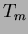have a common boundary (-dimensional face in),andare considered as neighbors. The notion of a set of `neighboring nodes' is generalized by the definition of natural-neighbor nodes. The natural neighbors of any node are those in the neighboring Voronoi cells, or equivalently, those to which the node is connected by the sides of the Delaunay triangle. The above definition extends if we are interested in finding the natural neighbors of any sampling point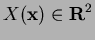. By including the sampling pointin the Delaunay triangulation, the natural neighbors ofare the set of nodes which are connected to it. It is noteworthy to point out that the number of natural neighbors is a function of position of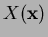, and depends on the local nodal density.

Consider an interpolation scheme for a function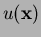:, in the form:(2)

where() are the function values at thenatural neighbors, andare the weights (shape functions in FE) associated with each node. In the context of natural neighbor interpolation, the weightsare taken as the n-n coordinates of the pointin the plane. Natural-neighbor coordinates were introduced by [1,2] and may be defined in any number of dimensions. To illustrate, we use an example indicated in . In Figure 1a, five nodes and the sides of the Voronoi cells are shown. If a pointis added in cell 3, then a new Voronoi cell can be placed around it (see Figure 1b). The n-n coordinates ofwith respect to a neighbor is defined as the ratio of the area of their overlapping Voronoi cells to the total area of the Voronoi cell about. For instance, in Figure 1b,is given by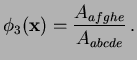(3)

The five overlapping regions in Figure 1b are known as second-order Voronoi cells, whileis a first-order Voronoi cell.By the above definition of, the following two properties are self-evident:(4)

Now, ifwere to coincide with any node, say nodefor instance, then it is readily seen thatand. Therefore the n-n coordinates satisfy the Kronecker-delta property:(5)

and hence Eq. (2) is an interpolation:.

Sinceis only non-zero in the union of thecircles that pass through the vertices of the Delaunay triangles about node, the n-n interpolation is a local (interpolating) scheme. In addition, theare continuosly differentiable (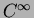) everywhere except at the nodes,, where all the derivatives are discontinuous . The proof of the above in one-dimension is trivial. In 1D, it is readily derived that theare precisely linear FE shape functions:,, where. Hence FEM is realized (by the n-n interpolation) in 1D, where it is well-known that the displacement derivative is discontinuous at nodes (element boundaries in 1D).

The natural neighbor coordinatesalso satisfies an important property known as the Local Coordinate Property (LCP) -- geometrical coordinates are interpolated exactly :(6)

Since the interpolant satisfies linear consistency, in the context of elastostatics, the approximation (trial function) can exactly represent rigid-body motion and linear displacement fields.

SubsectionsNext: Governing Equations and Weak Up: A Note on Natural Previous: Voronoi Diagrams and Delaunay
N. Sukumar